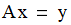# NMath User's Guide

Chapter 21. Least Squares Solutions (.NET, C#, CSharp, VB, Visual Basic, F#)

NMath includes least squares classes for solving the overdetermined linear system:In a linear model, a quantity y depends on one or more independent variables a1, a2,...,an such that y = x0 + x1a1 + ... + xnan. The goal of a least squares problem is to solve for the best values of x0,x1,...,xn. The least squares solution is the value of x that minimizes the residual vector ||Ax - y||.

NMath provides classes for:

ordinary least squares (OLS)

weighted least squares (WLS)

iteratively reweighted least squares (IRLS)

This chapter describes the NMath least square classes, and how to construct and use them.

Top

Top